1Simon Fraser University 2Autodesk AI Lab 3Shandong University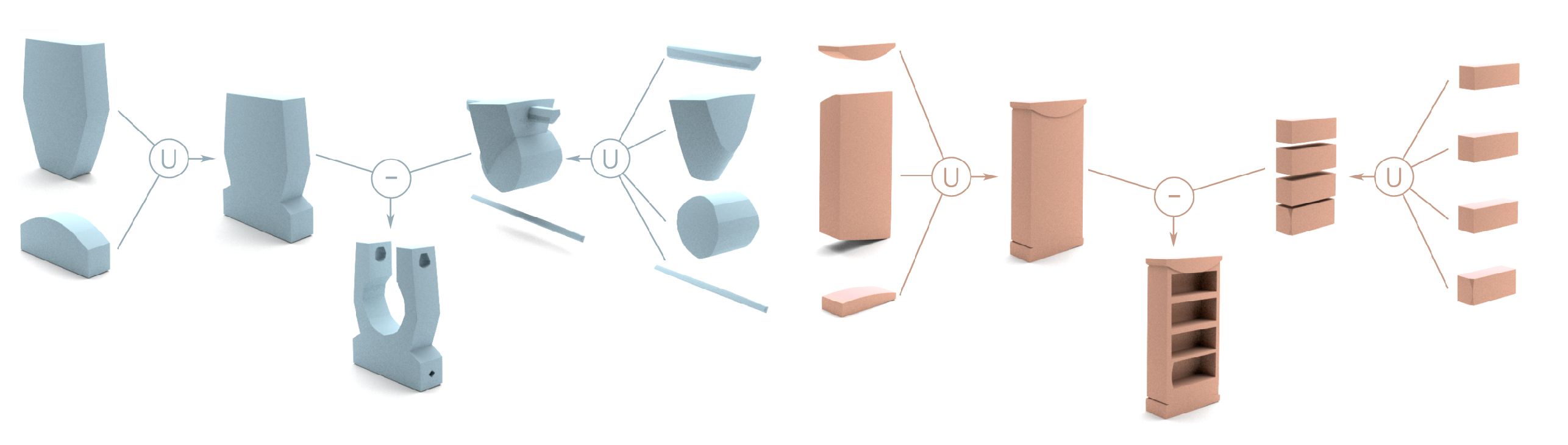Our network learns compact and interpretable implicit representations of 3D CAD shapes in the form of primitive assemblies via CSG operations, without any assembly supervision.

### Abstract

We introduce CAPRI-Net, a self-supervised neural network for learning compact and interpretable implicit representations of 3D computer-aided design (CAD) models, in the form of adaptive primitive assemblies. Given an input 3D shape, our network reconstructs it by an assembly of quadric surface primitives via constructive solid geometry (CSG) operations. Without any ground-truth shape assemblies, our self-supervised network is trained with a reconstruction loss, leading to faithful 3D reconstructions with sharp edges and plausible CSG trees. While the parametric nature of CAD models does make them more predictable locally, at the shape level, there is much structural and topological variation, which presents a significant generalizability challenge to state-of-the-art neural models for 3D shapes. Our network addresses this challenge by adaptive training with respect to each test shape, with which we finetune the network that was pre-trained on a model collection. We evaluate our learning framework on both ShapeNet and ABC, the largest and most diverse CAD dataset to date, in terms of reconstruction quality, sharp edges, compactness, and interpretability, to demonstrate superiority over current alternatives for neural CAD reconstruction.

### Method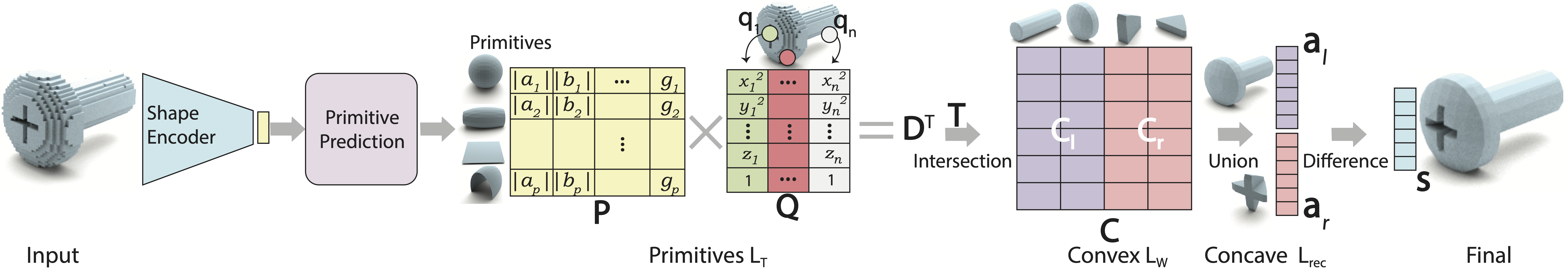Overview of our network. Given an input 3D shape as a point cloud or voxels, we first map it into a latent code using an encoder. This latent code is used to predict $$p$$ primitives with parameters included in $$\mathcal{P}$$. For any query point $$\mathcal{q}_j$$ packed in matrix $$\mathcal{Q}$$, we can obtain the matrix $$\mathcal{D}$$ indicating approximate signed distance from the query point to each primitive. A selection matrix $$\mathcal{T}$$ is used to select a small set of primitives from the primitive set to group convex shapes in matrix $$\mathcal{C}$$ which indicates inside/outside values for query points w.r.t convex shapes. Then, we perform min operation on each half of $$\mathcal{C}$$ (i.e. $$\mathcal{T}_{l}$$ and $$\mathcal{T}_{r}$$) to union convex shapes into two (possibly) concave shapes and get inside/outside indication vectors $$\mathcal{a}_{l}$$ and $$\mathcal{a}_{r}$$ for left and right concave shapes. Finally, we perform a difference operation as $$\mathcal{a}_{l} - \mathcal{a}_{r}$$ to obtain the final point-wise inside/outside indicator $$\mathcal{s}$$. $$L_\mathcal{T}$$, $$L_\mathcal{W}$$, and $$L_{rec}$$ are the loss functions we define for our network.

### Results

#### Reconstructed Mesh Quality

The output meshes from our method contain sharp edges and regular surfaces as expected from performing the CSG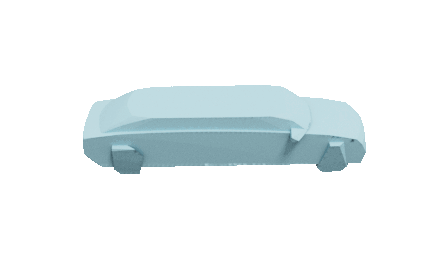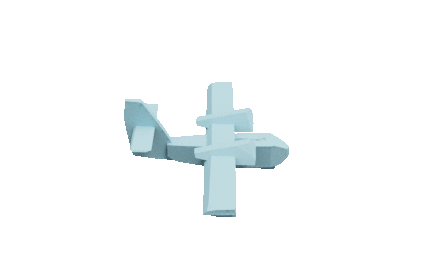#### Self-supervised learned CSG Tree

CSG-based CAD mesh outputting process of our method. We assemble primitives into convex shapes and perform CSG operations to output a CAD mesh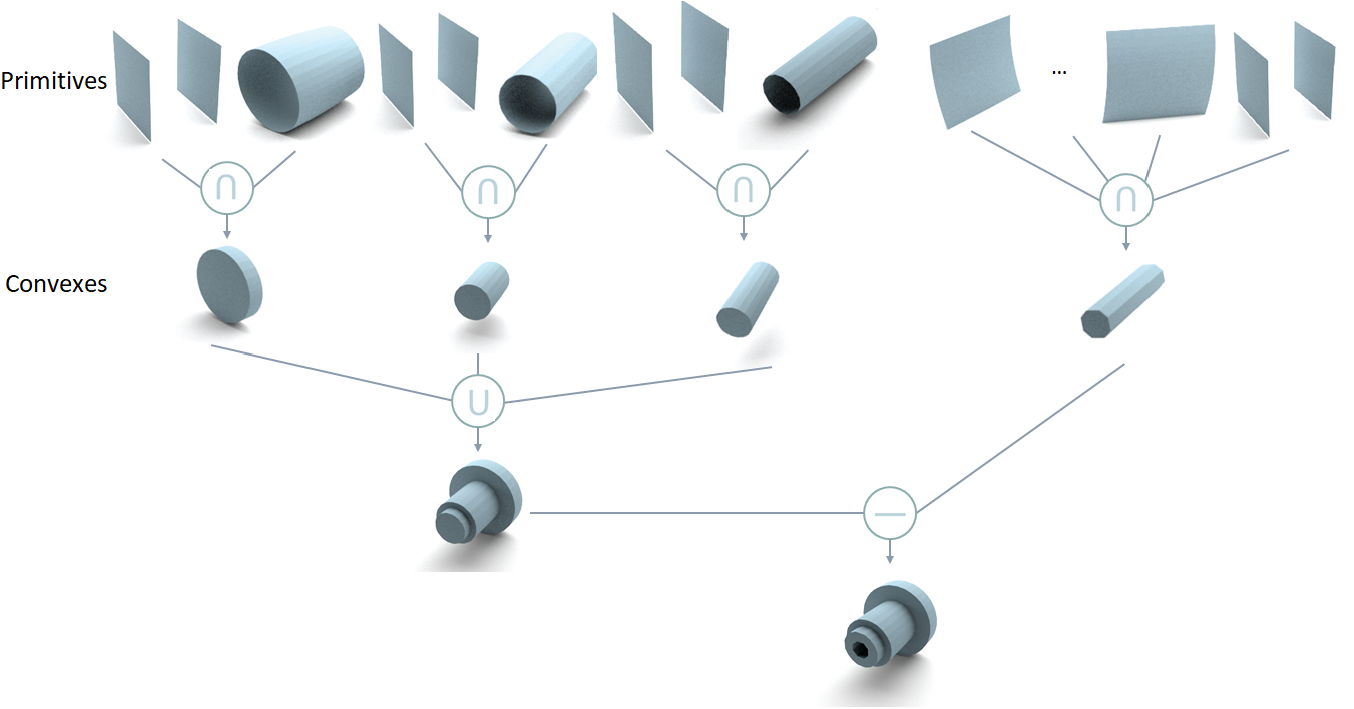#### Comparison on ABC Dataset

Visual comparisons between reconstruction results from point clouds (8,192 points) on ABC. Pay attention to the insets which show noticeable surface artifacts from IM-Net and SIREN results, both at $${128}^{3}$$ resolution.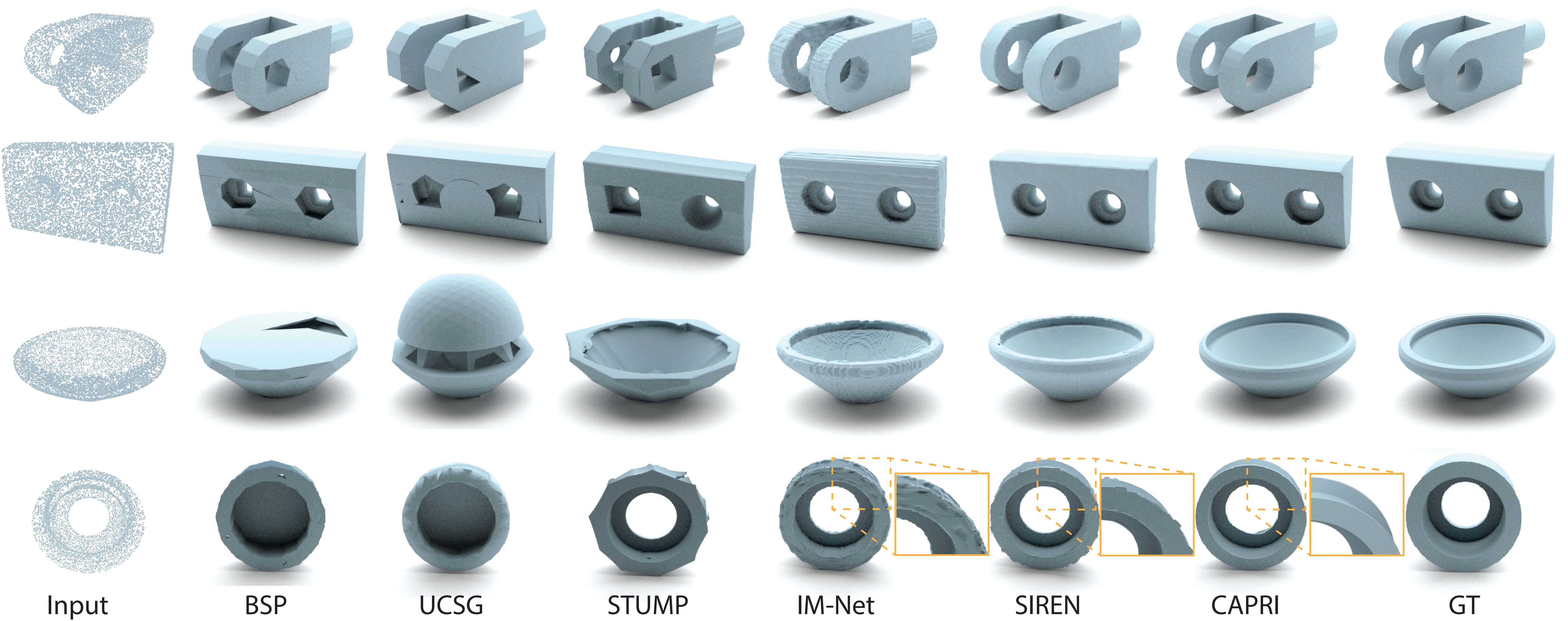#### Comparison on ShapeNet

Visual comparisons between reconstruction results from $${64}^{3}$$ voxel inputs on ABC. We also show number of surface primitives (#P) and number of convexes (#C) reconstructed. As UCSG and CSG-Stump do not produce surface primitives, only convex solids (e.g., boxes and spheres), we count the convexes.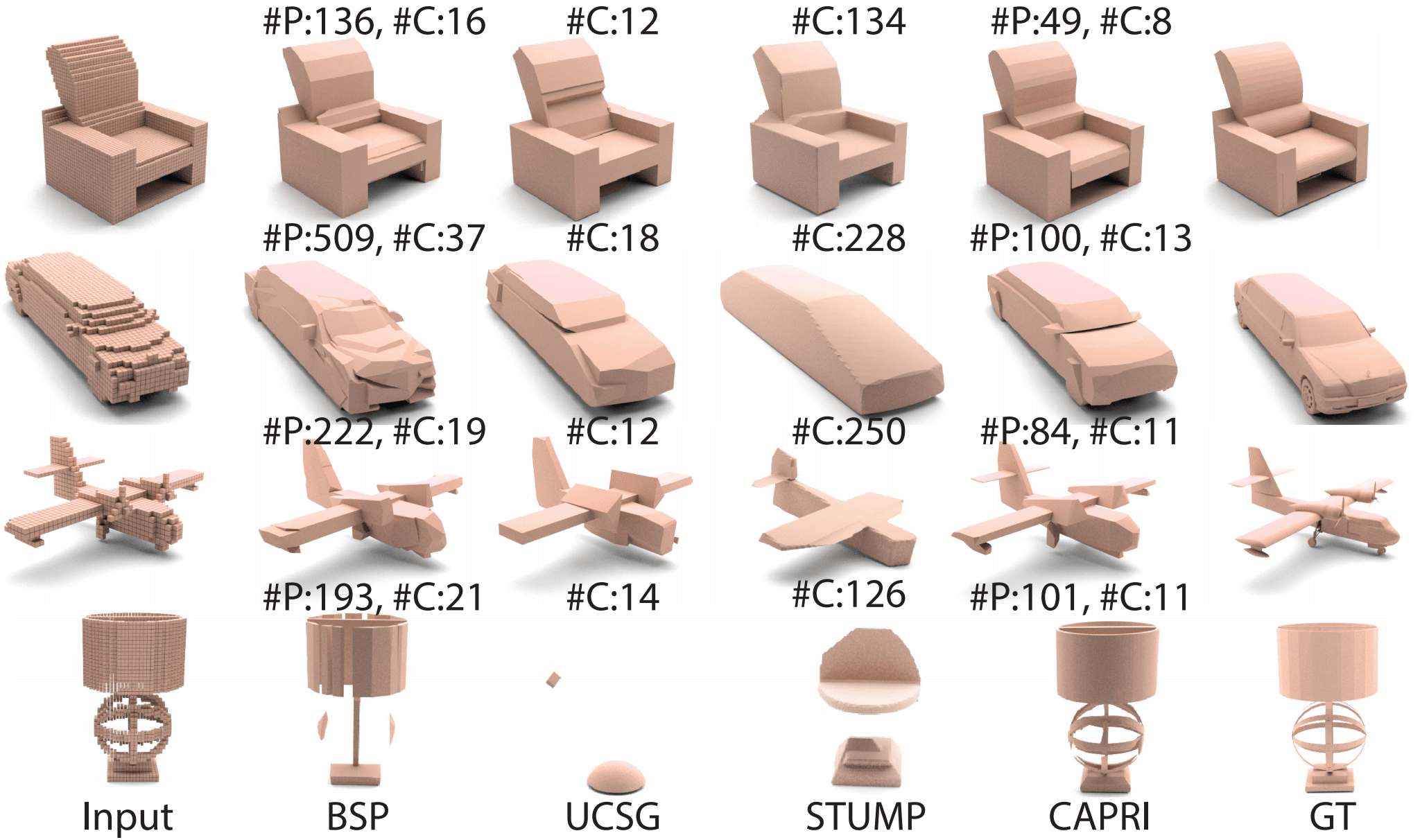### Citation

@InProceedings{Yu_2022_CVPR, author = {Yu, Fenggen and Chen, Zhiqin and Li, Manyi and Sanghi, Aditya and Shayani, Hooman and Mahdavi-Amiri, Ali and Zhang, Hao}, title = {CAPRI-Net: Learning Compact CAD Shapes With Adaptive Primitive Assembly}, booktitle = {Proceedings of the IEEE/CVF Conference on Computer Vision and Pattern Recognition (CVPR)}, month = {June}, year = {2022}, pages = {11768-11778} }